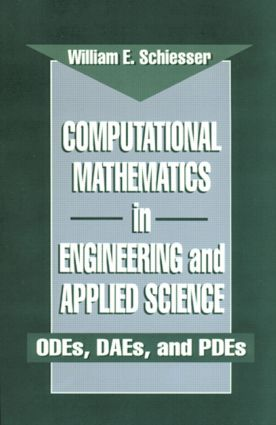Computational Mathematics in Engineering and Applied Science

ODEs, DAEs, and PDEs, 1st Edition

CRC Press

608 pages

Hardback: 9780849373732
pub: 1993-10-25
\$275.00
x
eBook (VitalSource) : 9780429156779
pub: 2014-07-22
from \$137.50

FREE Standard Shipping!

Description

Computational Mathematics in Engineering and Applied Science provides numerical algorithms and associated software for solving a spectrum of problems in ordinary differential equations (ODEs), differential algebraic equations (DAEs), and partial differential equations (PDEs) that occur in science and engineering. It presents detailed examples, each including a complete analysis of a computer code written in transportable Fortran 77. Each example also includes a discussion of the problem equations, the coding of the equations, and the computed numerical solution. The benefits of using quality general-purpose library routines to solve ODE/DAE/PDE problems are illustrated as well.

This popular, classic book is a valuable reference for methodologies in numerical mathematics applicable to a broad spectrum of problems encountered across many disciplines- virtually all fields of science and engineering. It also serves as an excellent text for senior undergraduates or beginning graduate students in computational science.

The General Problem in Ordinary and Partial Differential Equations

The Numerical Integration of Initial Value Ordinary Differential Equations

Partial Differential Equations First Order in Time

Partial Differential Equations Second and Zeroth Order in Time

Appendices:

A Second Order Adams Bashforth ODE Integrator

Spatial Differentiation Routines

Library of ODE/DAE/PDE Applications